# The Bias Variance Tradeoff and Regularization Slides by

• Slides: 44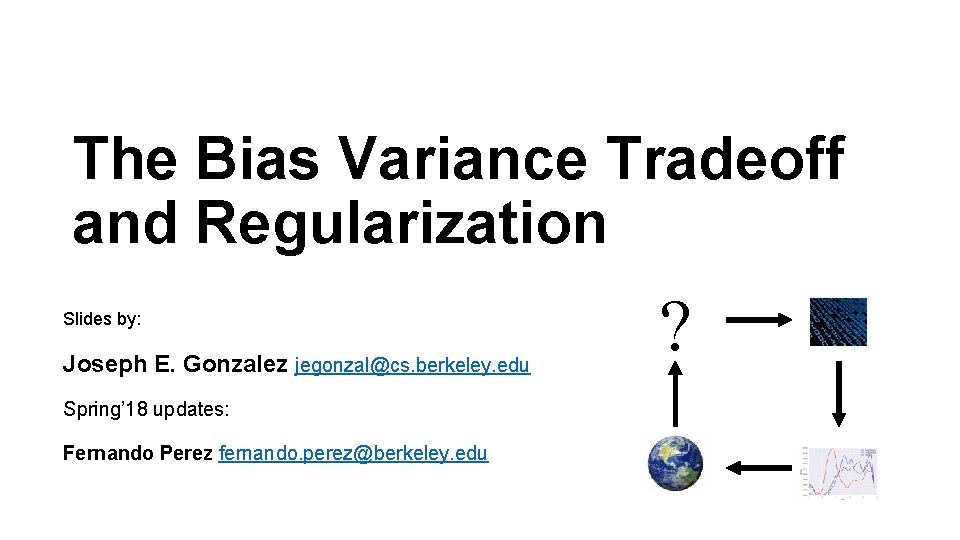The Bias Variance Tradeoff and Regularization Slides by: Joseph E. Gonzalez [email protected] berkeley. edu Spring’ 18 updates: Fernando Perez fernando. [email protected] edu ?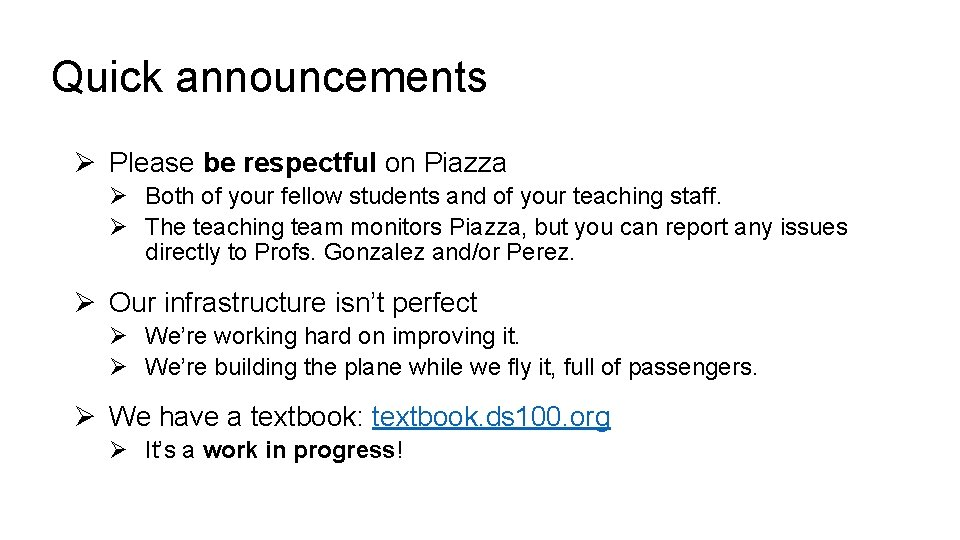Quick announcements Ø Please be respectful on Piazza Ø Both of your fellow students and of your teaching staff. Ø The teaching team monitors Piazza, but you can report any issues directly to Profs. Gonzalez and/or Perez. Ø Our infrastructure isn’t perfect Ø We’re working hard on improving it. Ø We’re building the plane while we fly it, full of passengers. Ø We have a textbook: textbook. ds 100. org Ø It’s a work in progress!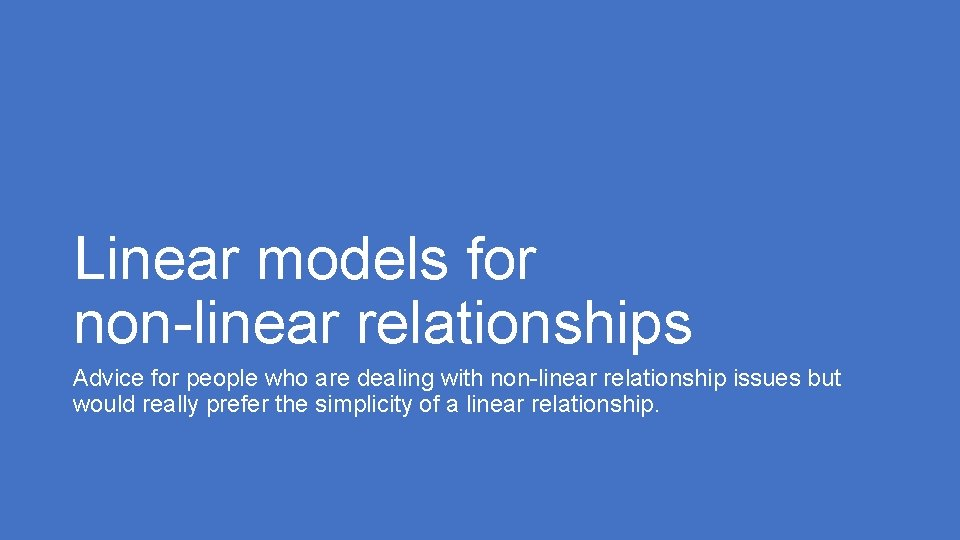Linear models for non-linear relationships Advice for people who are dealing with non-linear relationship issues but would really prefer the simplicity of a linear relationship.Is this data Linear? What does it mean to be linear?What does it mean to be a linear model? In what sense is the above model linear? Are linear models linear in the 1. the features? 2. the parameters?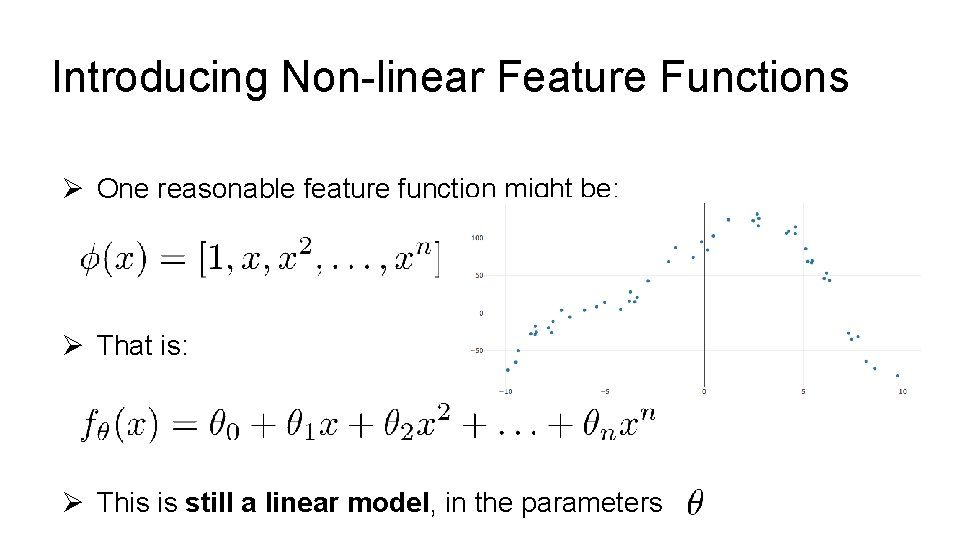Introducing Non-linear Feature Functions Ø One reasonable feature function might be: Ø That is: Ø This is still a linear model, in the parameters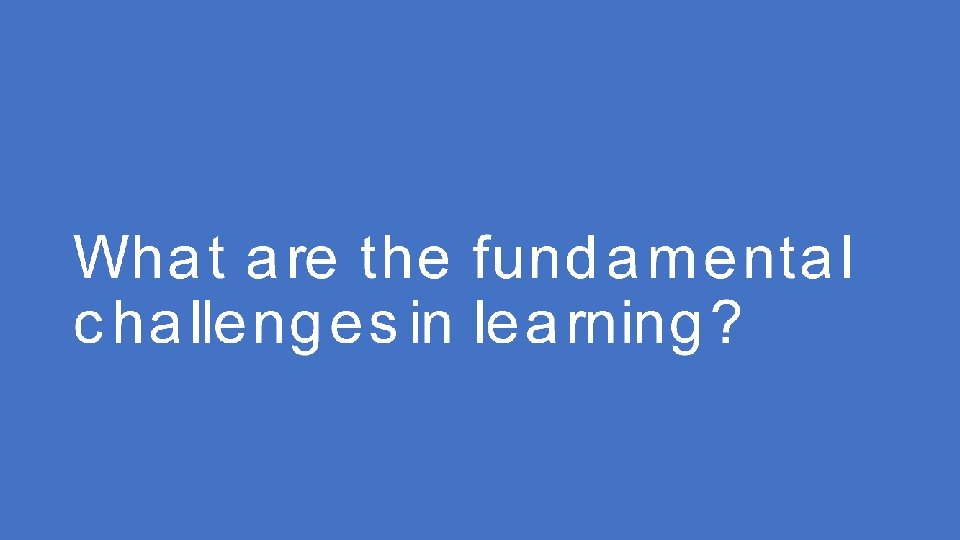What are the fundamental challenges in learning?Fundamental Challenges in Learning? Ø Fit the Data Ø Provide an explanation for what we observe Ø Generalize to the World Ø Predict the future Ø Explain the unobserved Is this cat grumpy or are we overfitting to human faces?Fundamental Challenges in Learning? Ø Bias: the expected deviation between the predicted value and the true value Ø Variance: two sources Ø Observation Variance: the variability of the random noise in the process we are trying to model. Ø Estimated Model Variance: the variability in the predicted value across different training datasets.Bias The expected deviation between the predicted value and the true value Ø Depends on both the: All possible functions Ø choice of f Ø learning procedure Ø Under-fitting Bias True FunctionObservation Variance the variability of the random noise in the process we are trying to model Ø measurement variability Ø stochasticity Ø missing information Beyond our control (usually)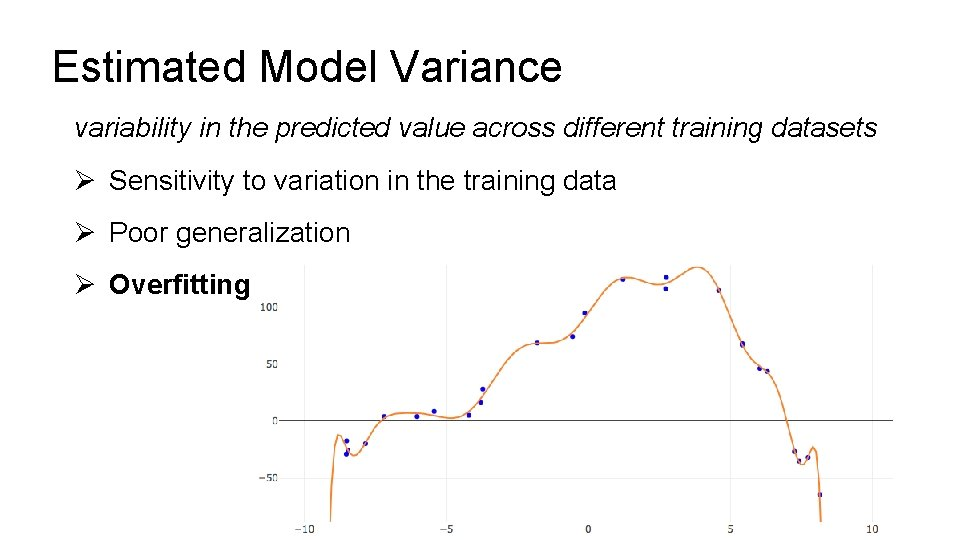Estimated Model Variance variability in the predicted value across different training datasets Ø Sensitivity to variation in the training data Ø Poor generalization Ø Overfitting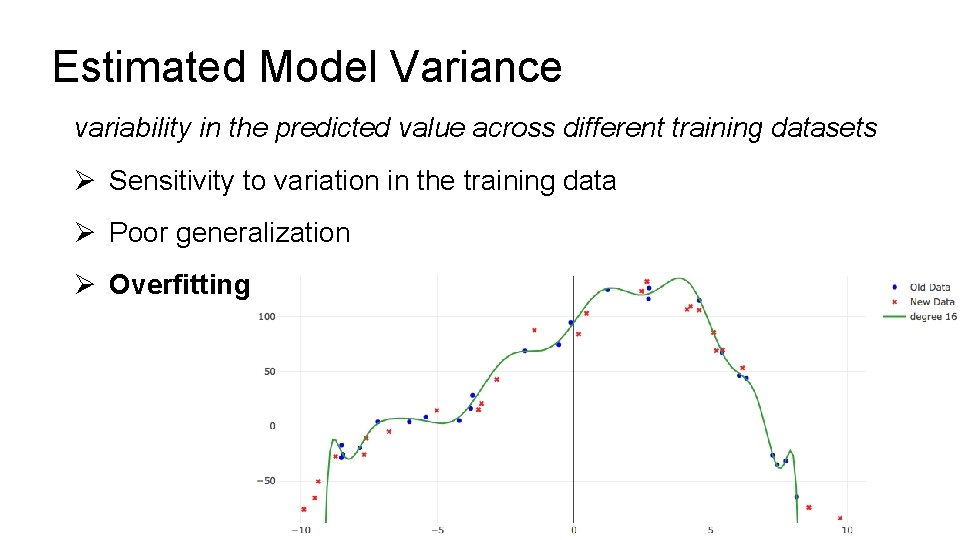Estimated Model Variance variability in the predicted value across different training datasets Ø Sensitivity to variation in the training data Ø Poor generalization Ø Overfitting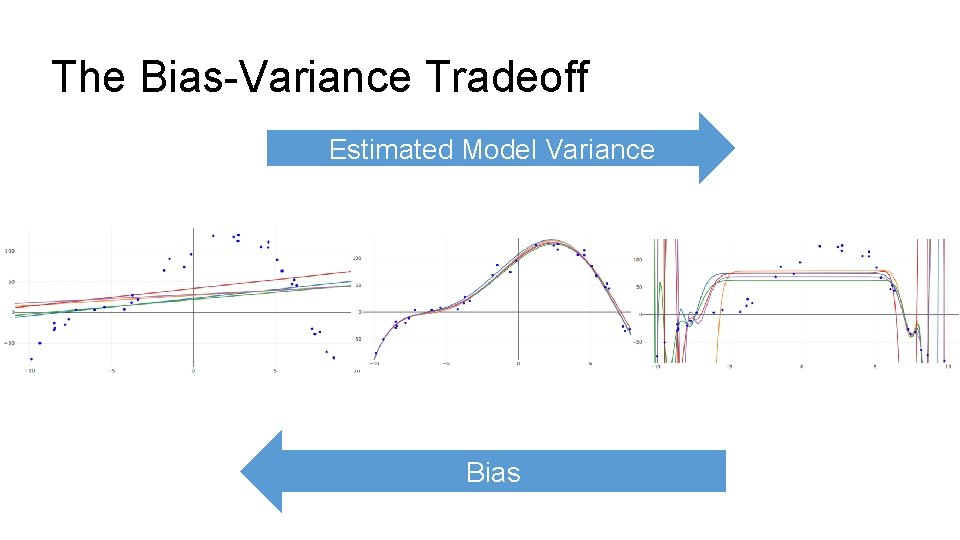The Bias-Variance Tradeoff Estimated Model Variance BiasVisually Ø The red center of the board: the true behavior of the universe. Ø Each dart: a specific function, that models the universe Ø Our parameters select these functions. . From Understanding the Bias-Variance Tradeoff, by Scott Fortmann-RoeDemo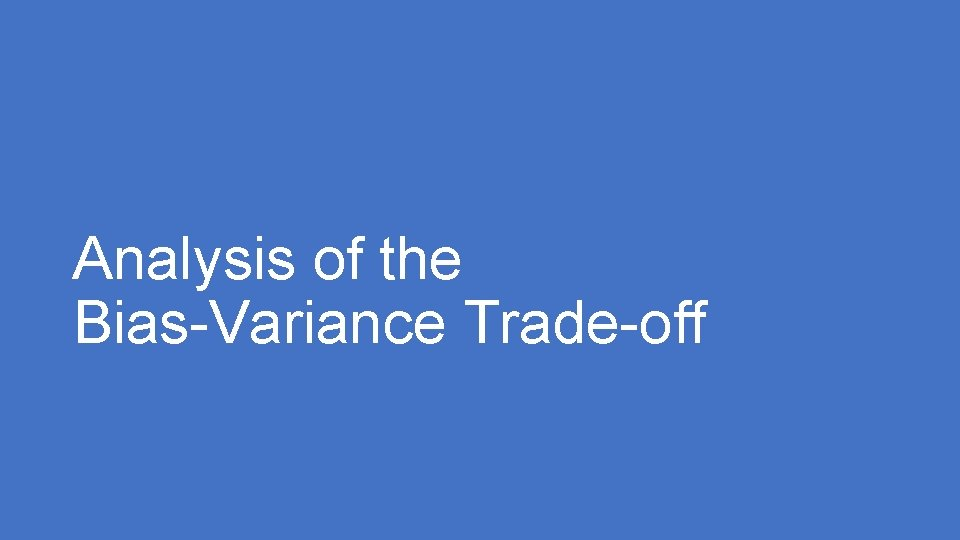Analysis of Squared Error Ø For the test point x the expected error: Ø Random variables are red Noise term: True Function Assume noisy observations y is a random variable Assume training data is random θ is a random variableAnalysis of Squared Error Goal: = Obs. Var. + 2 (Bias) + Mod. Var. 2 (Bias) + Variance Other terminology: “Noise” += Subtracting and adding h(x) Useful Eqns:= Expanding in terms of a and b: = Useful Eqns: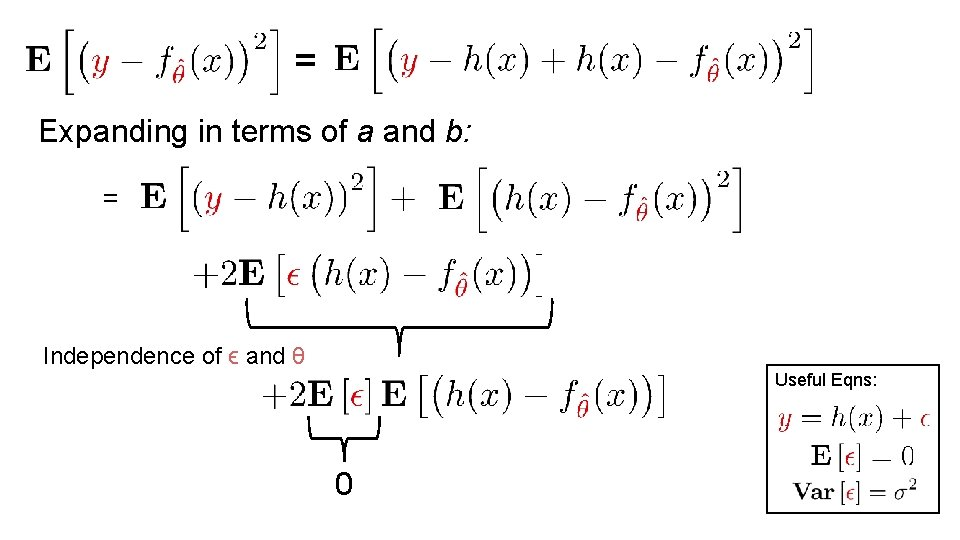= Expanding in terms of a and b: = Independence of ϵ and θ Useful Eqns: 0= = Obs. Value True Value Pred. Value Obs. Variance “Noise” Term Model Estimation Useful Eqns: Error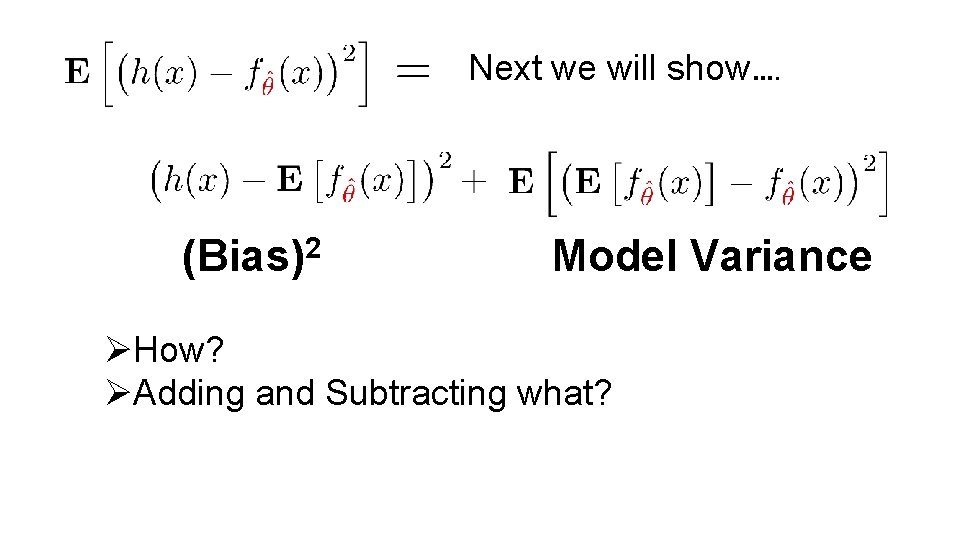Next we will show…. (Bias)2 Model Variance ØHow? ØAdding and Subtracting what?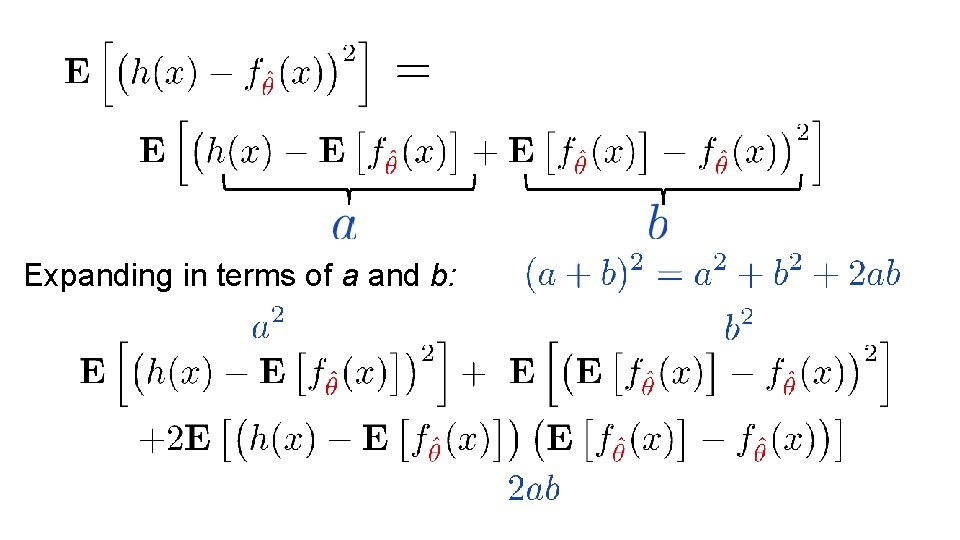Expanding in terms of a and b: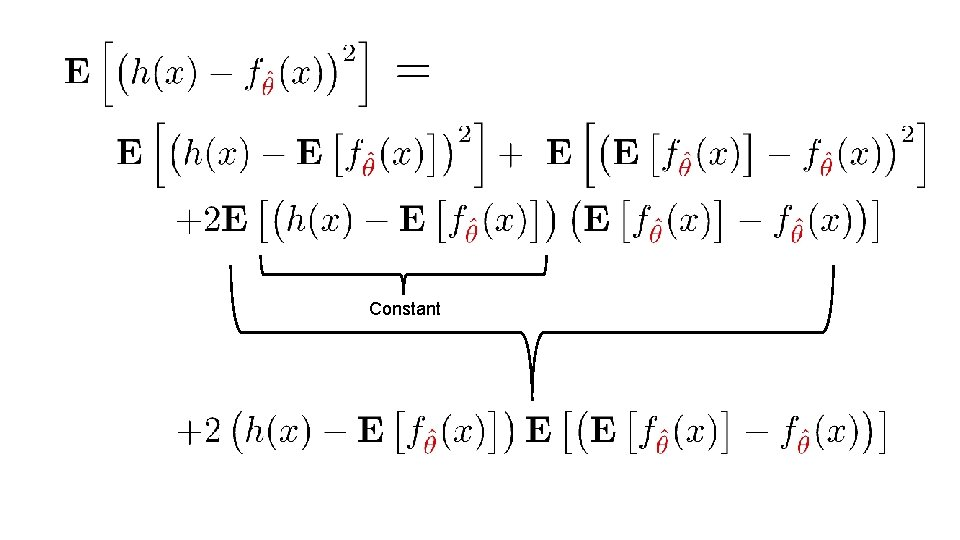Constant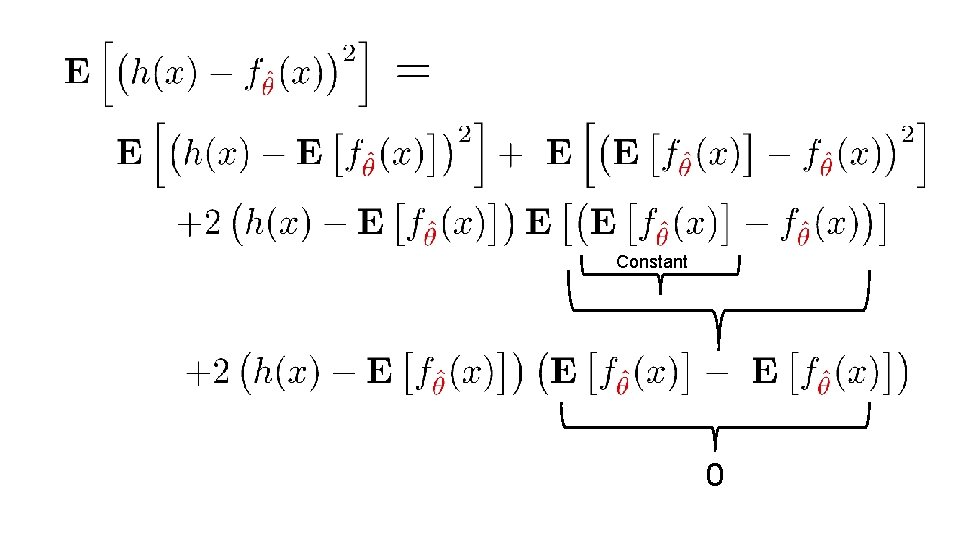Constant 0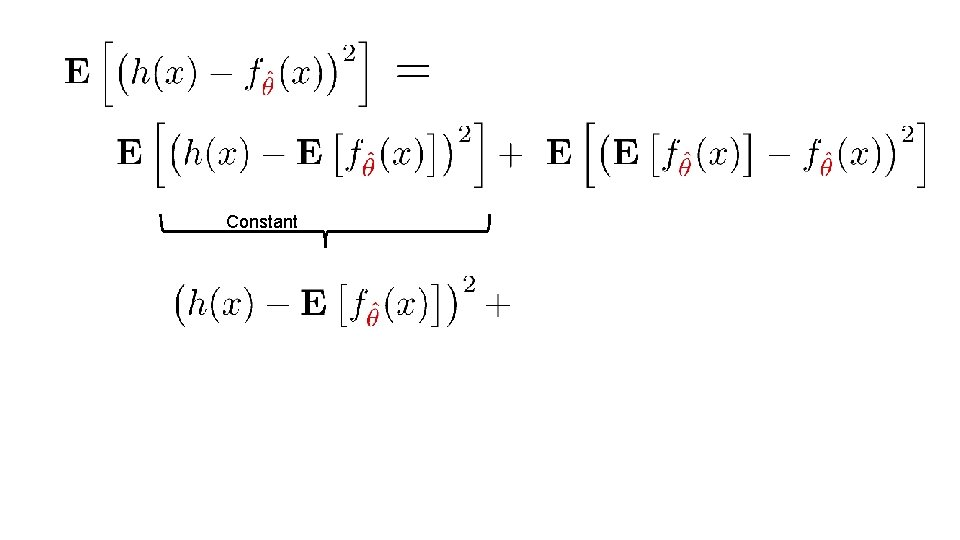Constant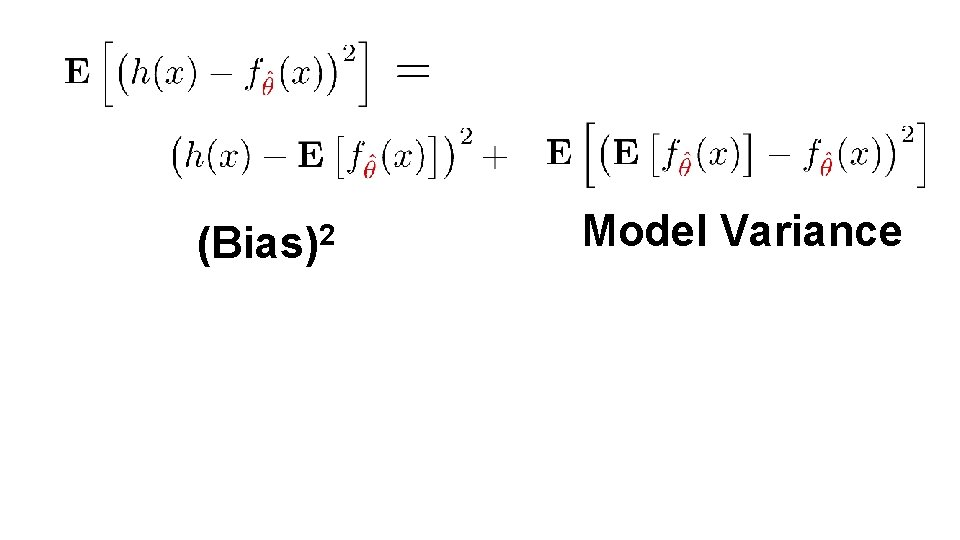(Bias)2 Model Variance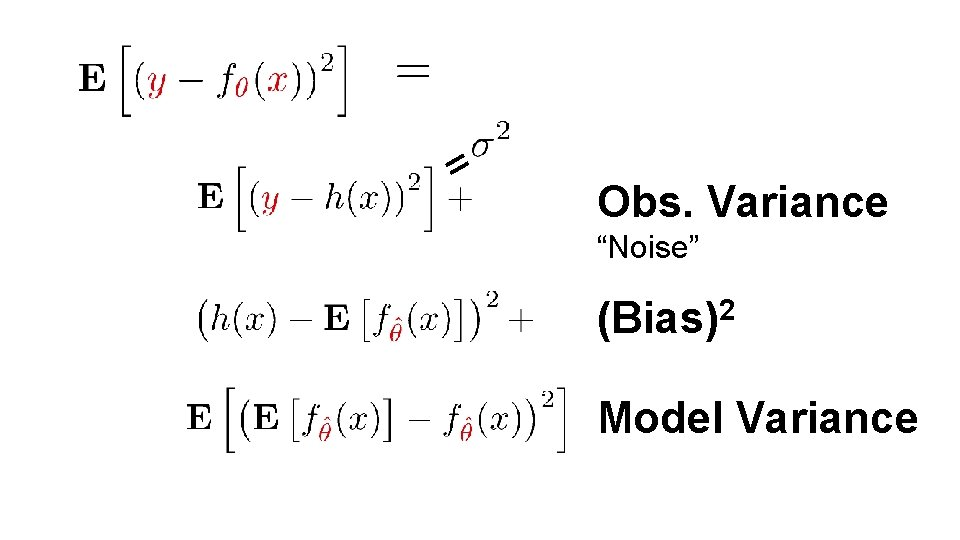= Obs. Variance “Noise” (Bias)2 Model Variance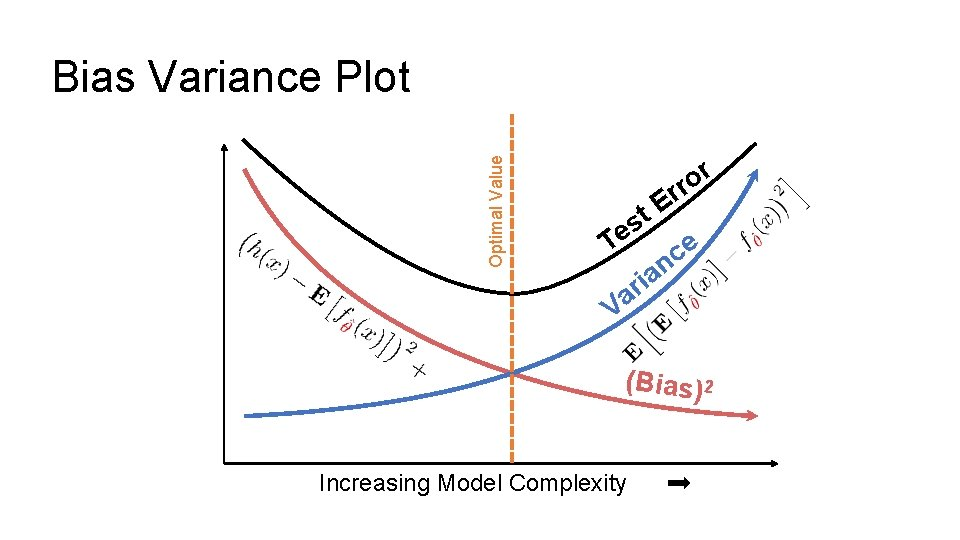Optimal Value Bias Variance Plot r o rr E t s e T e c n a i r Va (Bias) 2 Increasing Model Complexity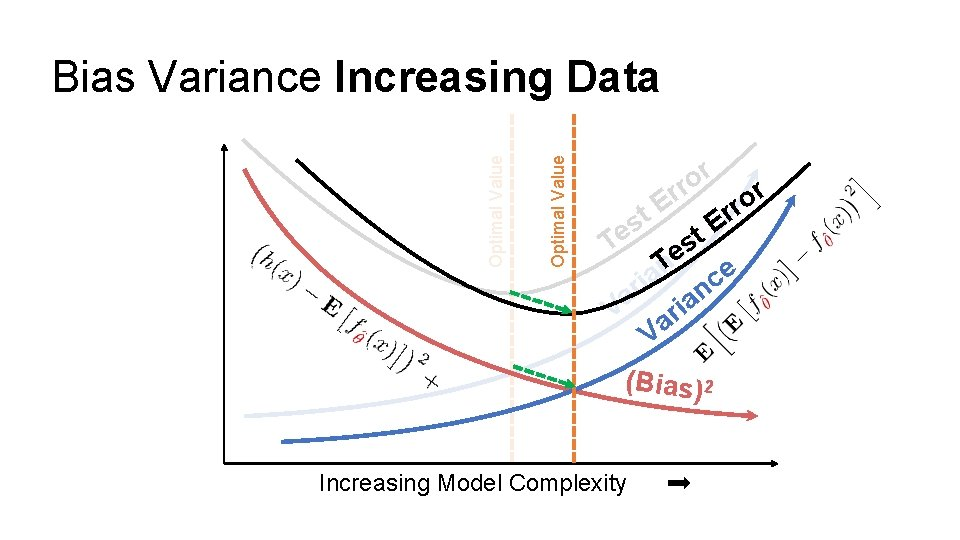Optimal Value Bias Variance Increasing Data r o rr r o E r r t s E e t T se e c Tn e a c i r n a a i V ar V (Bias) 2 Increasing Model ComplexityHow do we control model complexity? Ø Number of features Ø Choices of features Ø Next: Regularization Optimal Value Ø So far: r o rr E t s e T e c n a i r Va (Bias) 2 Increasing Model Complexity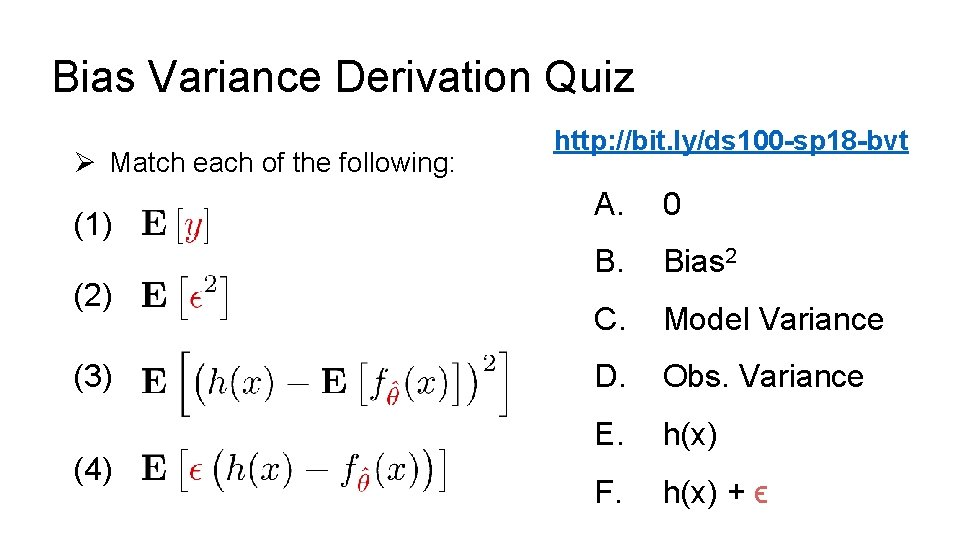Bias Variance Derivation Quiz Ø Match each of the following: (1) (2) (3) (4) http: //bit. ly/ds 100 -sp 18 -bvt A. 0 B. Bias 2 C. Model Variance D. Obs. Variance E. h(x) F. h(x) + ϵBias Variance Derivation Quiz Ø Match each of the following: (1) (2) (3) (4) http: //bit. ly/ds 100 -sp 18 -bvt A. 0 B. Bias 2 C. Model Variance D. Obs. Variance E. h(x) F. h(x) + ϵ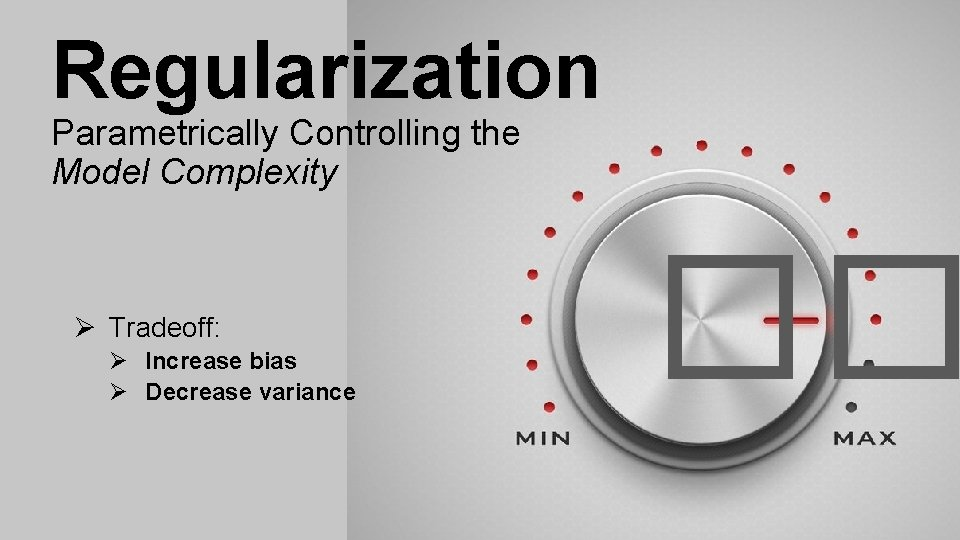Regularization Parametrically Controlling the Model Complexity Ø Tradeoff: Ø Increase bias Ø Decrease variance ��Basic Idea of Regularization Fit the Data Ø How should we define R(θ)? Ø How do we determine ��? Penalize Complex Models Regularization ParameterThe Regularization Function R(θ) Goal: Penalize model complexity Recall earlier: Ø More features overfitting … Ø How can we control overfitting through θ Ø Proposal: set weights = 0 to remove featuresCommon Regularization Functions Ridge Regression (L 2 -Reg) Ø Distributes weight across related features (robust) Ø Analytic solution (easy to compute) Ø Does not encourage sparsity small but non-zero weights. LASSO (L 1 -Reg) Ø Encourages sparsity by setting weights = 0 Ø Used to select informative features Ø Does not have an analytic solution numerical methodsRegularization and Norm Balls s Los �� 2 � t �op �� 1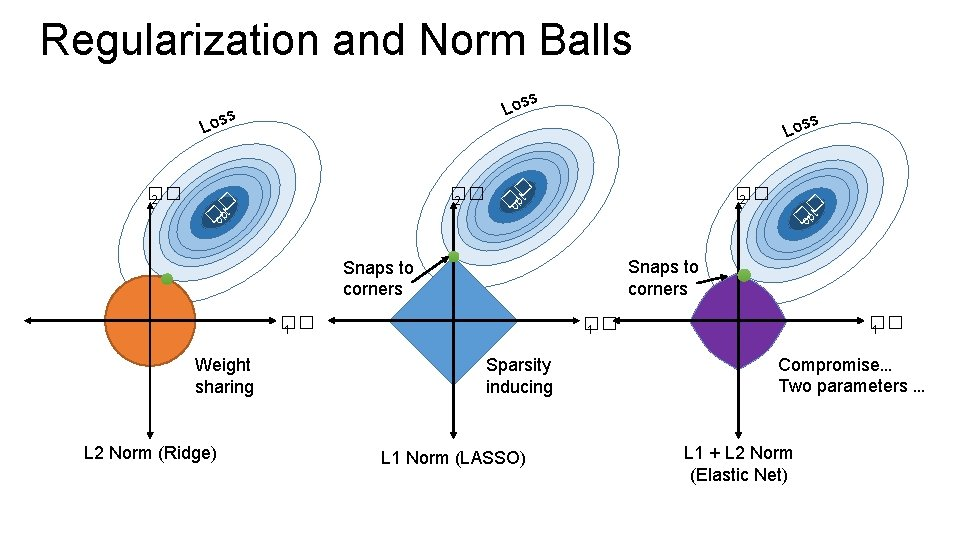Regularization and Norm Balls s Los �� 2 � t �op s Los � t �op �� 2 Snaps to corners �� 1 Weight sharing L 2 Norm (Ridge) � t �op �� 1 Sparsity inducing L 1 Norm (LASSO) Compromise… Two parameters … L 1 + L 2 Norm (Elastic Net)Python Demo! The shapes of the norm balls. Maybe show reg. effects on actual models.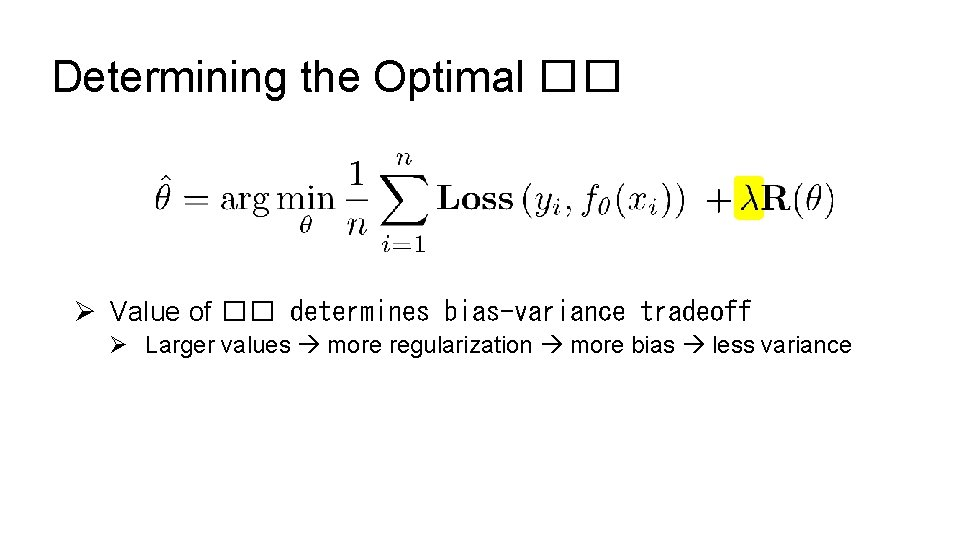Determining the Optimal �� Ø Value of �� determines bias-variance tradeoff Ø Larger values more regularization more bias less variance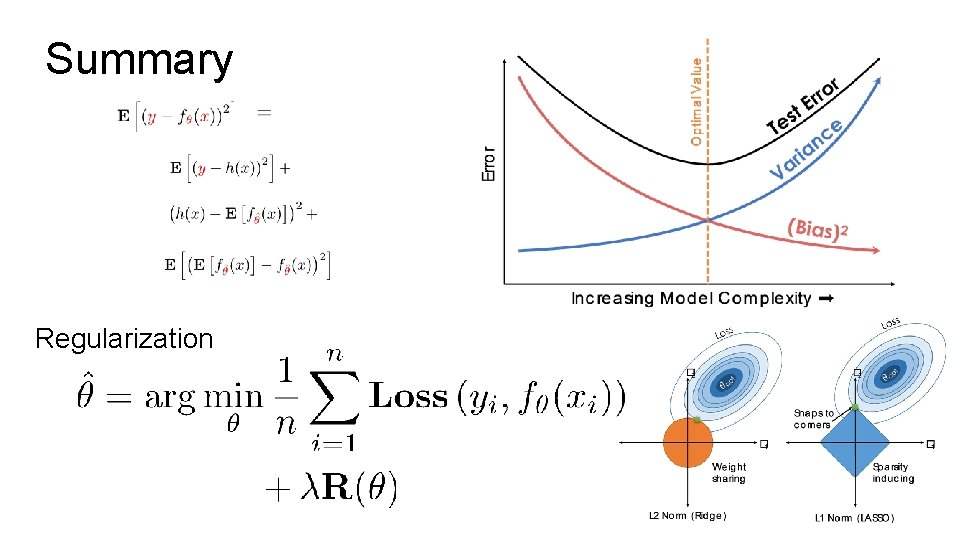Summary Regularization